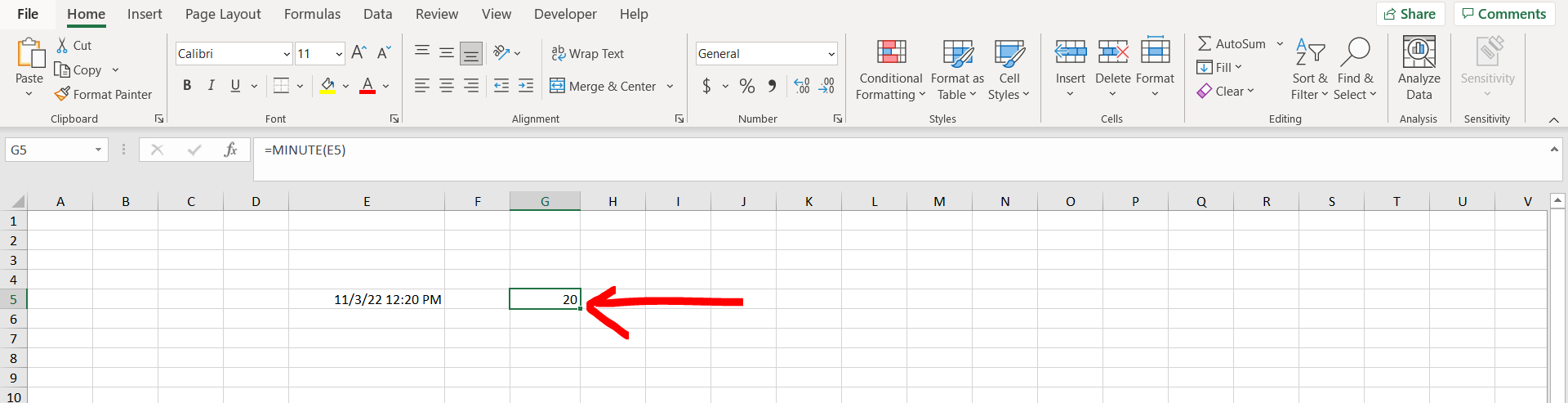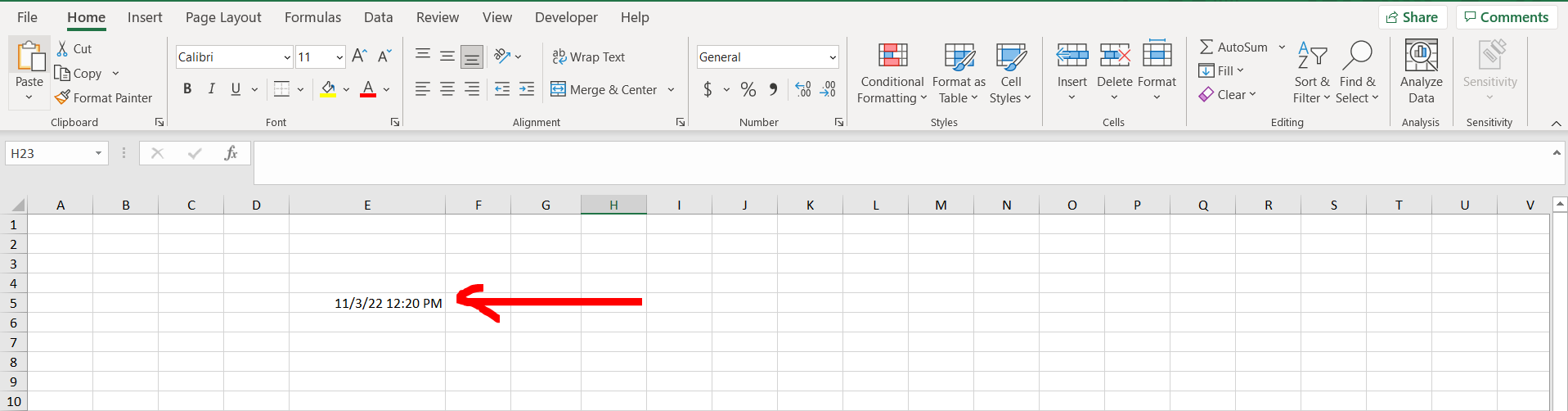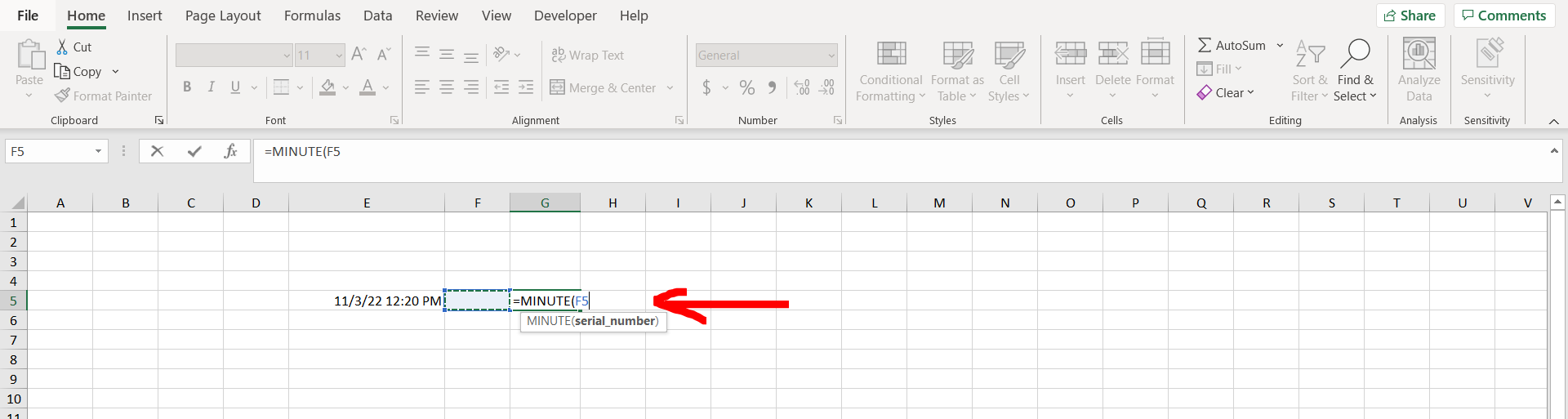# How to calculate minutes in ExcelExcel offers a very interesting way to calculate minutes. We can cater to this problem statement by using the “=MINUTE()” formula. We can perform the below mentioned way to calculate difference between two dates and times in excel:

### Step 1 – Excel workbook with a time– Open the desired Excel workbook which contains time in a cell

### Step 2 – Minute formula applied– Now type the formula “=MINUTE(“ and give the reference of the cell where the time is present, and hit Enter.

### Step 3 – Minutes calculated– We can see that the minutes have been extracted from the cell.